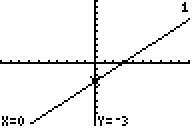Knowledge Base

Solution 11962: Graphing a Function on the TI-83 Family or TI-84 Plus Family Graphing Calculator.

How do I graph a function on a TI-83 family or TI-84 Plus family graphing calculator?

The function graphing mode will graph equations in the format of y in terms of x. Therefore, equations will need to be solved in terms of y before being entered into the unit. The example below will demonstrate how to graph a function on a TI-83 family or TI-84 Plus family graphing calculator.

Example:
Graph y - x = -3

Solution:
First, solve for y. This is done manually using algebra.

The new equation is: y=x-3

To graph the equation:
1) Press [Y=]
2) Input x - 3 on the top line after Y1=
3) Press [GRAPH]

The graph will now be displayed on the screen.Please refer to the TI-83 family or TI-84 Plus family graphing calculator guidebooks for additional information.